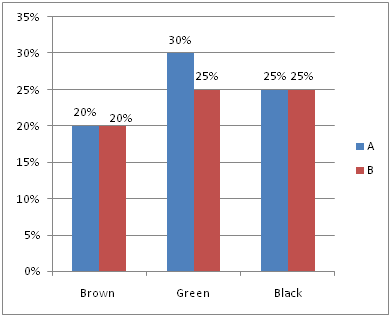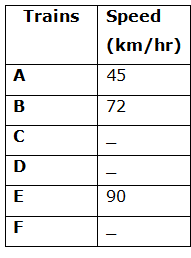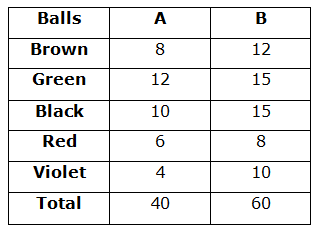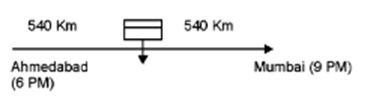# SBI PO Quantitative Aptitude Questions 2019 (Day-20) High Level New Pattern

SBI PO 2019 Notification is about to come and it is the most awaited exam among the aspirants. We all know that new pattern questions are introducing every year in the SBI PO exam. Further, the questions are getting tougher and beyond the level of the candidate’s expectations.

Our IBPS Guide is providing High-Level New Pattern Quantitative Aptitude Questions for SBI PO 2019 so the aspirants can practice it on a daily basis. These questions are framed by our skilled experts after understanding your needs thoroughly. Aspirants can practice these high-level questions daily to familiarize with the exact exam pattern. We wish that your rigorous preparation leads you to a successful target of becoming SBI PO.

[WpProQuiz 5186]

### Click Here for SBI PO Pre 2019 High-Quality Mocks Exactly on SBI Standard

Directions (1 – 5): Study the following graph carefully and answer the given questions.

The bar graph shows the probability percent of randomly drawing a different colour balls (Brown, green, black, violet and red) from two bags A and B.Note: 1: Total number of violet colour balls in bag A is 4 and total number of red colour balls in bag B is 8.

Note 2: Probability of drawing a red colour ball from bag A is 3/20.

Note 3: Probability of drawing a violet colour ball from bag B is 1/6.

1) If x black balls are taken out from bag B and put into bag A and now the probability of selecting a black ball from bag A is 1/3, then find the probability of selecting a green ball from bag B after transferring?

a) 2/11

b) 3/11

c) 5/11

d) 4/11

e) 7/11

2) If one ball from each bag is drawn at random, then what is the probability of the event, that one brown ball is from bag A and one violet ball is from bag B?

a) 1/8

b) 1/25

c) 1/24

d) 1/30

e) 1/40

3) If one ball from each bag is drawn at random, the probability of red ball from bag A is what percentage of the probability of brown ball from bag B?

a) 75%

b) 45%

c) 50%

d) 60%

e) 80%

4) What is the ratio of probability of drawing two violet colour balls from bag B at random to the probability of drawing two violet colour balls from bag A at random?

a) 59: 195

b) 121: 171

c) 171: 78

d) 129: 59

e) None of these

5) If x black and 2x green colour balls are taken out from bag A and put into bag B, and now probability of selecting a green colour ball from bag B is 1/3. Find the value of ‘x’?

a) 6

b) 2

c) 4

d) 3

e) 5

Directions (6 – 10): Study the following graph carefully and answer the given questions.

The table shows the speed of different trains in km/hr.6) Train B started at 9.00 am from station X towards Y. After two hours, Train E started from station Y towards X. The two trains are expected to cross each other at 1.30 pm. Owing to a signal problem arising at 12 noon, the speed of each of them was reduced by the same quantity and they crossed each other at 4.30 pm. What is the new speed of train started from station X?

a) 36 km/hr

b) 54 km/hr

c) 18 km/hr

d) 24 km/hr

e) 27 km/hr

7) 300 m length of Train A passes point P completely. At the same time, a motorbike starts from point P with the speed of 70 km/h. When it exactly reaches the middle point of the train A, the train increases its speed to 60 km/h and motorbike reduces its speed to 65 km/h. How much distance will the motorbike travel while passing the train completely?

a) 2.45 km

b) 2.54 km

c) 3.12 km

d) 2.37 km

e) None of these

8) Train C’s journey is disrupted due to an accident on its track after it has travelled 30 km. Its speed then comes down to 4/5th of its original and consequently it runs 45 min late. Had the accident taken place 18 km farther away, it would have been 36 min late. The original speed of the train C is what percentage of the speed of train E?

a) 200/3 %

b) 100/3 %

c) 50/3 %

d) 190/3 %

e) 250/3 %

9) Train D departs from Ahmadabad at 6 pm for Bombay. At 9 pm a train F, whose average speed exceeds that of the train D by 15 km/h, leaves Bombay for Ahmadabad. Two trains meet each other mid-route. At what time do they meet, given that the distance between the cities is 1080 km?

Statement I:  400 m length of the train D crosses a pole in 32 sec.

Statement II: 600 m length of train F crosses a pole in 36 sec.

a) Only I

b) Only II

c) Either I or II

d) Both I and II

e) Neither I nor II

10) Quantity I: If 4.5 km length of the train D crosses a pole in 6 minutes, then find the speed of the train D?

Quantity II: If 6 km length of the train F crosses a pole in 6 minutes, then find the speed of the train F?

a) Quantity I > Quantity II

b) Quantity I < Quantity II

c) Quantity I ≥ Quantity II

d) Quantity I ≤ Quantity II

e) Quantity I = Quantity II (or) Relationship cannot be determined

Directions (1 – 5):

Total number of balls in bag A = x

Total number of balls in bag B = y

Probability percentage of drawing a red colour ball from bag A

= 3/20 * 100

= 15%

Probability percentage of drawing a violet colour ball from bag A

= 100% – (20% + 30% + 25% + 15%)

= 100% – 90%

= 10%

Ratio of the number of Brown, Green, Black, Red and Violet colour balls in bag A,

= 20: 30: 25: 15: 10

= 4: 6: 5: 3: 2

Number of violet colour balls in bag A = 2/(20) * Total number of balls in bag A

4 = 2/20 * x

= > x = 40 balls

Probability percentage of drawing a violet colour ball from bag B

= 1/6 * 100

= 50/3 %

Probability percentage of drawing a red colour ball from bag B

= 100% – (20% + 25% + 25% + 50/3%)

= [300% – (60%+75%+75%+50%)]/3

= (300% – 260%)/3

= 40/3 %

Ratio of the number of Brown, Green, Black, Red and Violet colour balls in bag B,

= 20: 25: 25: 40/3: 50/3

= 60: 75: 75: 40: 50

= 12: 15: 15: 8: 10

Number of red colour balls in bag B = 8/(60) * Total number of balls in bag B

8 = 8/60 * y

= > y = 8 * 60/8

= > y = 60 ballsNumber of black balls in bag A = 10 + x

Total number of balls in bag A = 40 + x

Probability of drawing a black ball from bag A = (10+x)C1 / (40+x)C1 = 1/3

(10+x) / (40+x) = 1/3

30 + 3x = 40 + x

= > 2x = 10 = > x = 5

Total number of balls in bag B after taking 5 black balls

= 60 – 5 = 55

Required probability = 15C1/55C1

= 15/55 = 3/11

Probability of getting a brown ball from bag A = 8C1/40C1

= 8/40 = 1/5

Probability of getting a violet ball from bag B = 10C1/60C1

= 10/60 = 1/6

Required probability = 1/5 * 1/6 = 1/30

Probability of getting a red ball from bag A = 6C1/40C1

= 6/40 = 3/20

Probability of getting a brown ball from bag B = 12C1/60C1

= 12/60 = 1/5

Required percentage = (3/20)/(1/5) *100

= 3/20 * 5 * 100

= 75%

Probability of drawing two violet colour balls from bag B

= 10C2/60C2

Probability of drawing two violet colour balls from bag A

= 4C2/40C2

Required ratio = 10C2/60C2 : 4C2/40C2

= (10*9)/(60*59) : (4*3)/(40*39)

= (3*5*13) : (59)

= 195 : 59

Number of black colour balls in bag B = 15 + x

Number of green colour balls in bag B = 15 + 2x

Total number of balls in bag B = 60 + 3x

Probability of selecting a green colour ball from bag B = 1/3

= > (15+2x)C1/(60+3x)C1 = 1/3

(15 + 2x)/(60 + 3x) = 1/3

45 + 6x = 60 + 3x

= > 3x = 15

= > x = 5

Direction (6-10) :

Speed of train B = 72 km/hr

Speed of train E = 90 km/hr

Distance covered by train B at 11.00 am = 2 * 72 = 144 km

Total distance covered by train B and train E at 1.30 pm

= (72 + 90) * (11.00 am – 1.30 pm)

= 162 * (2 hours 30 minutes)

= 162 * (2.5)

= 405 km

Total distance between X and Y = 144 + 405 = 549 km

Owing to a signal problem arising at 12 noon,

Distance covered by Train B and Train E at 12 noon

= 2* 72 + (72 + 90)

= 144 + 162 = 306

Remaining distance = 549 – 306

= 243 km

If 243 km distance covered by two trains at 4.30 pm

Total time = (4.30 pm – 12 noon) = 4 hours 30 minutes

New speed = 243/(4.5) = 54 km/hr

Train B and Train E reduced same quantity of speed,

Let us take the reduced speed be x,

(72 – x) + (90 – x) = 54

162 – 2x = 54

162 – 54 = 2x

108 = 2x

X = 108/2 = 54 km/hr

New speed of train B = 72 – 54 = 18 km/hr

Speed of train A = 45 km/hr = 45 * 5/18 = 12.5 m/s

Speed of motor bike = 70 km/hr = 70 * 5/18 m/s

Time taken by motor bike to reach at the mid-point of the train

= 150 / (70 * 5/18 – 45 * 5/18)

= 150 * 18/5 * (1/25)

= 21.6 sec

New speed of train = 60 * 5/18

New speed of bike = 65 * 5/18

Time taken by motor bike to reach remaining 150 m

= 150/[(65 – 60)*5/18]

= 150/5 * 18/5

= 108 sec

Total distance will the motorbike travel while passing the train completely

= 21.6 * 70 * 5/18 + 108 * 65 * 5/18

= 420 + 1950

= 2370 m

= 2.37 km

Let us take the speed of train C be x km/hr,

According to the question,

18/(4x/5) – 18/x = (45 – 36)/60

(18*5)/4x – 18/x = 9/60

90/4x – 72/4x = 9/60

18/4x = 3/20

= > x = 30 km/hr

Required percentage = 30/90 * 100

= 1/3*100

= 100/3 %

Let us take the speed of train D be x km/hr and the speed of train F be (x+15) km/hr

Distance covered by train D and train F is 540 km each

Time taken by train D = 540/x

Time taken by train F = 540/(x+15)From I,

Speed of train D = 400/32 = 12.5 sec = 12.5 * 18/5

= 45 km/hr

Time taken by train D to cover 540 km

= 540/45 = 12 hours

Train D and train F will meet (6 pm + 12 hours) = 6 am

Statement I alone is sufficient to answer the question.

From II,

Speed of train F = 600/36 = 50/3 m/s = 50/3 * 18/5

= 60 km/hr

Time taken by train F to cover 540 km

= 540/60 = 9 hours

Train F and train D will meet after (9 pm + 9 hours) = 6 am

Statement II alone is sufficient to answer the question.

From I and II, either of the statement is sufficient to answer the question.

Quantity I: If 4.5 km length of the train D crosses a pole in 6 minutes, then find the speed of the train D

Speed of the train D = 4.5/(6/60)

= 4.5 * 60/6 = 45 km/hr

Quantity II: If 6 km length of the train F crosses a pole in 6 minutes, then find the speed of the train F

Speed of the train F = 6/(6/60)

= 6 *60/6 = 60 km/hr

Quantity I < Quantity II

### Click Here for SBI PO Pre 2019 High-Quality Mocks Exactly on SBI Standard